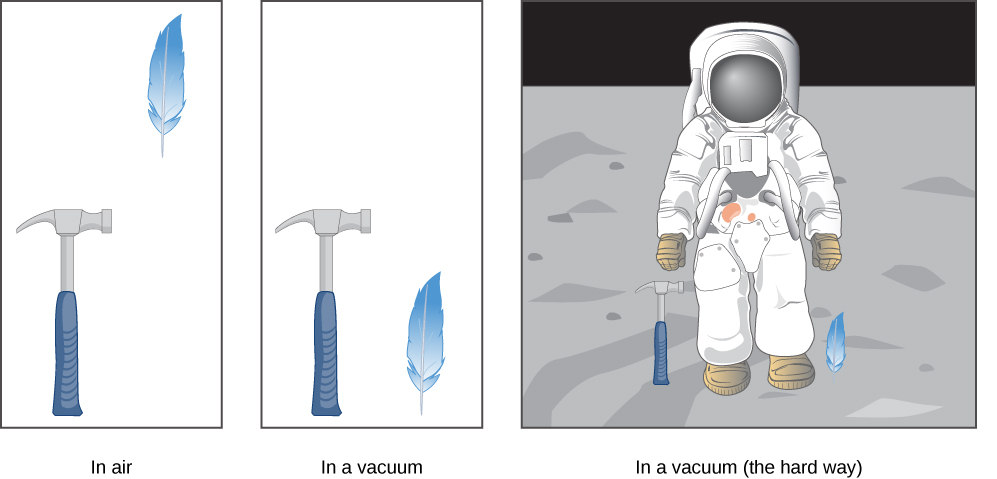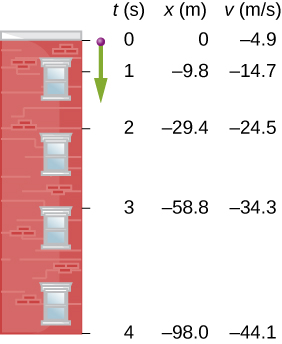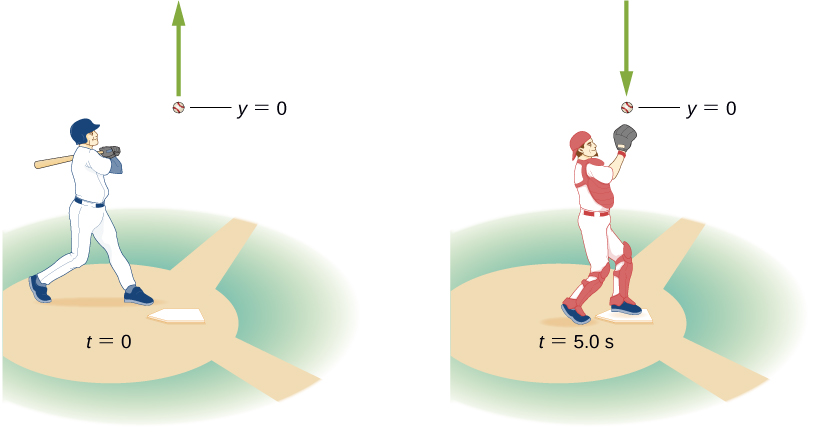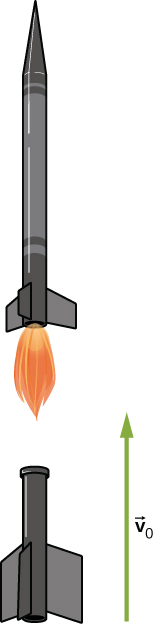Motion Along a Straight Line

# Free Fall

### Learning Objectives

By the end of this section, you will be able to:

• Use the kinematic equations with the variables y and g to analyze free-fall motion.
• Describe how the values of the position, velocity, and acceleration change during a free fall.
• Solve for the position, velocity, and acceleration as functions of time when an object is in a free fall.

An interesting application of (Figure) through (Figure) is called free fall, which describes the motion of an object falling in a gravitational field, such as near the surface of Earth or other celestial objects of planetary size. Let’s assume the body is falling in a straight line perpendicular to the surface, so its motion is one-dimensional. For example, we can estimate the depth of a vertical mine shaft by dropping a rock into it and listening for the rock to hit the bottom. But “falling,” in the context of free fall, does not necessarily imply the body is moving from a greater height to a lesser height. If a ball is thrown upward, the equations of free fall apply equally to its ascent as well as its descent.

### Gravity

The most remarkable and unexpected fact about falling objects is that if air resistance and friction are negligible, then in a given location all objects fall toward the center of Earth with the same constant acceleration, independent of their mass. This experimentally determined fact is unexpected because we are so accustomed to the effects of air resistance and friction that we expect light objects to fall slower than heavy ones. Until Galileo Galilei (1564–1642) proved otherwise, people believed that a heavier object has a greater acceleration in a free fall. We now know this is not the case. In the absence of air resistance, heavy objects arrive at the ground at the same time as lighter objects when dropped from the same height (Figure).

A hammer and a feather fall with the same constant acceleration if air resistance is negligible. This is a general characteristic of gravity not unique to Earth, as astronaut David R. Scott demonstrated in 1971 on the Moon, where the acceleration from gravity is only 1.67 m/s2 and there is no atmosphere.In the real world, air resistance can cause a lighter object to fall slower than a heavier object of the same size. A tennis ball reaches the ground after a baseball dropped at the same time. (It might be difficult to observe the difference if the height is not large.) Air resistance opposes the motion of an object through the air, and friction between objects—such as between clothes and a laundry chute or between a stone and a pool into which it is dropped—also opposes motion between them.

For the ideal situations of these first few chapters, an object falling without air resistance or friction is defined to be in free fall. The force of gravity causes objects to fall toward the center of Earth. The acceleration of free-falling objects is therefore called acceleration due to gravity. Acceleration due to gravity is constant, which means we can apply the kinematic equations to any falling object where air resistance and friction are negligible. This opens to us a broad class of interesting situations.

Acceleration due to gravity is so important that its magnitude is given its own symbol, g. It is constant at any given location on Earth and has the average value

$g=9.81\phantom{\rule{0.2em}{0ex}}{\text{m/s}}^{2}\phantom{\rule{0.5em}{0ex}}\left(\text{or}\phantom{\rule{0.2em}{0ex}}32.2\phantom{\rule{0.2em}{0ex}}{\text{ft/s}}^{2}\right).$

Although g varies from 9.78 m/s2 to 9.83 m/s2, depending on latitude, altitude, underlying geological formations, and local topography, let’s use an average value of 9.8 m/s2 rounded to two significant figures in this text unless specified otherwise. Neglecting these effects on the value of g as a result of position on Earth’s surface, as well as effects resulting from Earth’s rotation, we take the direction of acceleration due to gravity to be downward (toward the center of Earth). In fact, its direction defines what we call vertical. Note that whether acceleration a in the kinematic equations has the value +g or −g depends on how we define our coordinate system. If we define the upward direction as positive, then $a=\text{−}g=-9.8\phantom{\rule{0.2em}{0ex}}{\text{m/s}}^{2},$ and if we define the downward direction as positive, then $a=g=9.8\phantom{\rule{0.2em}{0ex}}{\text{m/s}}^{2}$.

### One-Dimensional Motion Involving Gravity

The best way to see the basic features of motion involving gravity is to start with the simplest situations and then progress toward more complex ones. So, we start by considering straight up-and-down motion with no air resistance or friction. These assumptions mean the velocity (if there is any) is vertical. If an object is dropped, we know the initial velocity is zero when in free fall. When the object has left contact with whatever held or threw it, the object is in free fall. When the object is thrown, it has the same initial speed in free fall as it did before it was released. When the object comes in contact with the ground or any other object, it is no longer in free fall and its acceleration of g is no longer valid. Under these circumstances, the motion is one-dimensional and has constant acceleration of magnitude g. We represent vertical displacement with the symbol y.

Kinematic Equations for Objects in Free Fall

We assume here that acceleration equals −g (with the positive direction upward).

$v=\text{​}{v}_{0}-gt$
$y={y}_{0}+{v}_{0}t-\frac{1}{2}g{t}^{2}$
${v}^{2}={v}_{0}^{2}-2g\left(y-{y}_{0}\right)$
Problem-Solving Strategy: Free Fall
1. Decide on the sign of the acceleration of gravity. In (Figure) through (Figure), acceleration g is negative, which says the positive direction is upward and the negative direction is downward. In some problems, it may be useful to have acceleration g as positive, indicating the positive direction is downward.
2. Draw a sketch of the problem. This helps visualize the physics involved.
3. Record the knowns and unknowns from the problem description. This helps devise a strategy for selecting the appropriate equations to solve the problem.
4. Decide which of (Figure) through (Figure) are to be used to solve for the unknowns.

Free Fall of a Ball(Figure) shows the positions of a ball, at 1-s intervals, with an initial velocity of 4.9 m/s downward, that is thrown from the top of a 98-m-high building. (a) How much time elapses before the ball reaches the ground? (b) What is the velocity when it arrives at the ground?

The positions and velocities at 1-s intervals of a ball thrown downward from a tall building at 4.9 m/s.Strategy
Choose the origin at the top of the building with the positive direction upward and the negative direction downward. To find the time when the position is −98 m, we use (Figure), with ${y}_{0}=0,{v}_{0}=-4.9\phantom{\rule{0.2em}{0ex}}\text{m/s,}\phantom{\rule{0.2em}{0ex}}\text{and}\phantom{\rule{0.2em}{0ex}}g=9.8\phantom{\rule{0.2em}{0ex}}{\text{m/s}}^{2}$.

Solution

1. Substitute the given values into the equation:
$\begin{array}{}\\ \\ y={y}_{0}+{v}_{0}t-\frac{1}{2}g{t}^{2}\hfill \\ -98.0\phantom{\rule{0.2em}{0ex}}\text{m}=0-\left(4.9\phantom{\rule{0.2em}{0ex}}\text{m/s}\right)t-\frac{1}{2}\left(9.8\phantom{\rule{0.2em}{0ex}}{\text{m/s}}^{2}\right){t}^{2}.\hfill \end{array}$

This simplifies to

${t}^{2}+t-20=0.$

This is a quadratic equation with roots $t=-5.0\mathrm{s}\phantom{\rule{0.2em}{0ex}}\text{and}\phantom{\rule{0.2em}{0ex}}t=4.0\mathrm{s}$. The positive root is the one we are interested in, since time $t=0$ is the time when the ball is released at the top of the building. (The time $t=-5.0\mathrm{s}$ represents the fact that a ball thrown upward from the ground would have been in the air for 5.0 s when it passed by the top of the building moving downward at 4.9 m/s.)

2. Using (Figure), we have
$v={v}_{0}-gt=-4.9\phantom{\rule{0.2em}{0ex}}\text{m/s}-\left(9.8{\text{m/s}}^{2}\right)\left(4.0\phantom{\rule{0.2em}{0ex}}\text{s}\right)=-44.1\phantom{\rule{0.2em}{0ex}}\text{m/s}\text{.}$

Significance
For situations when two roots are obtained from a quadratic equation in the time variable, we must look at the physical significance of both roots to determine which is correct. Since $t=0$ corresponds to the time when the ball was released, the negative root would correspond to a time before the ball was released, which is not physically meaningful. When the ball hits the ground, its velocity is not immediately zero, but as soon as the ball interacts with the ground, its acceleration is not g and it accelerates with a different value over a short time to zero velocity. This problem shows how important it is to establish the correct coordinate system and to keep the signs of g in the kinematic equations consistent.

Vertical Motion of a Baseball
A batter hits a baseball straight upward at home plate and the ball is caught 5.0 s after it is struck (Figure). (a) What is the initial velocity of the ball? (b) What is the maximum height the ball reaches? (c) How long does it take to reach the maximum height? (d) What is the acceleration at the top of its path? (e) What is the velocity of the ball when it is caught? Assume the ball is hit and caught at the same location.

A baseball hit straight up is caught by the catcher 5.0 s later.Strategy
Choose a coordinate system with a positive y-axis that is straight up and with an origin that is at the spot where the ball is hit and caught.

Solution

1. (Figure) gives
$y={y}_{0}+{v}_{0}t-\frac{1}{2}g{t}^{2}$
$0=0+{v}_{0}\left(5.0\phantom{\rule{0.2em}{0ex}}\text{s}\right)-\frac{1}{2}\left(9.8\phantom{\rule{0.2em}{0ex}}\text{m}\text{/}{\text{s}}^{2}\right){\left(5.0\phantom{\rule{0.2em}{0ex}}\text{s}\right)}^{2},$

which gives ${v}_{0}=24.5\phantom{\rule{0.2em}{0ex}}\text{m/sec}$.

2. At the maximum height, $v=0$. With ${v}_{0}=24.5\phantom{\rule{0.2em}{0ex}}\text{m/s}$, (Figure) gives
${v}^{2}={v}_{0}^{2}-2g\left(y-{y}_{0}\right)$
$0={\left(24.5\phantom{\rule{0.2em}{0ex}}\text{m/s}\right)}^{2}-2\left(9.8{\text{m/s}}^{2}\right)\left(y-0\right)$

or

$y=30.6\phantom{\rule{0.2em}{0ex}}\text{m}\text{.}$
3. To find the time when $v=0$, we use (Figure):
$v={v}_{0}-gt$
$0=24.5\phantom{\rule{0.2em}{0ex}}\text{m/s}-\left(9.8{\text{m/s}}^{2}\right)t.$

This gives $t=2.5\phantom{\rule{0.2em}{0ex}}\text{s}$. Since the ball rises for 2.5 s, the time to fall is 2.5 s.

4. The acceleration is 9.8 m/s2 everywhere, even when the velocity is zero at the top of the path. Although the velocity is zero at the top, it is changing at the rate of 9.8 m/s2 downward.
5. The velocity at $t=5.0\mathrm{s}$ can be determined with (Figure):
$\begin{array}{cc}\hfill v& ={v}_{0}-gt\hfill \\ & =24.5\phantom{\rule{0.2em}{0ex}}\text{m/s}-9.8{\text{m/s}}^{2}\left(5.0\phantom{\rule{0.2em}{0ex}}\text{s}\right)\hfill \\ & =-24.5\phantom{\rule{0.2em}{0ex}}\text{m/s}.\hfill \end{array}$

Significance
The ball returns with the speed it had when it left. This is a general property of free fall for any initial velocity. We used a single equation to go from throw to catch, and did not have to break the motion into two segments, upward and downward. We are used to thinking of the effect of gravity is to create free fall downward toward Earth. It is important to understand, as illustrated in this example, that objects moving upward away from Earth are also in a state of free fall.

Check Your Understanding A chunk of ice breaks off a glacier and falls 30.0 m before it hits the water. Assuming it falls freely (there is no air resistance), how long does it take to hit the water? Which quantity increases faster, the speed of the ice chunk or its distance traveled?

It takes 2.47 s to hit the water. The quantity distance traveled increases faster.

Rocket Booster
A small rocket with a booster blasts off and heads straight upward. When at a height of $5.0\phantom{\rule{0.2em}{0ex}}\text{km}$ and velocity of 200.0 m/s, it releases its booster. (a) What is the maximum height the booster attains? (b) What is the velocity of the booster at a height of 6.0 km? Neglect air resistance.

A rocket releases its booster at a given height and velocity. How high and how fast does the booster go?Strategy
We need to select the coordinate system for the acceleration of gravity, which we take as negative downward. We are given the initial velocity of the booster and its height. We consider the point of release as the origin. We know the velocity is zero at the maximum position within the acceleration interval; thus, the velocity of the booster is zero at its maximum height, so we can use this information as well. From these observations, we use (Figure), which gives us the maximum height of the booster. We also use (Figure) to give the velocity at 6.0 km. The initial velocity of the booster is 200.0 m/s.

Solution

1. From (Figure), ${v}^{2}={v}_{0}^{2}-2g\left(y-{y}_{0}\right)$. With $v=0\phantom{\rule{0.2em}{0ex}}\text{and}\phantom{\rule{0.2em}{0ex}}{y}_{0}=0$, we can solve for y:
$y=\frac{{v}_{0}^{2}}{-2g}=\frac{\left(2.0\phantom{\rule{0.2em}{0ex}}×\phantom{\rule{0.2em}{0ex}}{10}^{2}\text{m}\text{/}{\text{s}\right)}^{2}}{-2\left(9.8\phantom{\rule{0.2em}{0ex}}\text{m}\text{/}{\text{s}}^{2}\right)}=2040.8\phantom{\rule{0.2em}{0ex}}\text{m}\text{.}$

This solution gives the maximum height of the booster in our coordinate system, which has its origin at the point of release, so the maximum height of the booster is roughly 7.0 km.

2. An altitude of 6.0 km corresponds to$y=1.0\phantom{\rule{0.2em}{0ex}}×\phantom{\rule{0.2em}{0ex}}{10}^{3}\phantom{\rule{0.2em}{0ex}}\text{m}$ in the coordinate system we are using. The other initial conditions are${y}_{0}=0,\phantom{\rule{0.2em}{0ex}}\text{and}\phantom{\rule{0.2em}{0ex}}{v}_{0}=200.0\phantom{\rule{0.2em}{0ex}}\text{m/s}$.

We have, from (Figure),

${v}^{2}={\left(200.0\phantom{\rule{0.2em}{0ex}}\text{m}\text{/}\text{s}\right)}^{2}-2\left(9.8\phantom{\rule{0.2em}{0ex}}\text{m}\text{/}{\text{s}}^{2}\right)\left(1.0\phantom{\rule{0.2em}{0ex}}×\phantom{\rule{0.2em}{0ex}}{10}^{3}\phantom{\rule{0.2em}{0ex}}\text{m}\right)⇒v=±142.8\phantom{\rule{0.2em}{0ex}}\text{m}\text{/}\text{s}.$

Significance
We have both a positive and negative solution in (b). Since our coordinate system has the positive direction upward, the +142.8 m/s corresponds to a positive upward velocity at 6000 m during the upward leg of the trajectory of the booster. The value v = −142.8 m/s corresponds to the velocity at 6000 m on the downward leg. This example is also important in that an object is given an initial velocity at the origin of our coordinate system, but the origin is at an altitude above the surface of Earth, which must be taken into account when forming the solution.

Visit this site to learn about graphing polynomials. The shape of the curve changes as the constants are adjusted. View the curves for the individual terms (for example, y = bx) to see how they add to generate the polynomial curve.

### Summary

• An object in free fall experiences constant acceleration if air resistance is negligible.
• On Earth, all free-falling objects have an acceleration g due to gravity, which averages $g=9.81\phantom{\rule{0.2em}{0ex}}{\text{m/s}}^{2}$.
• For objects in free fall, the upward direction is normally taken as positive for displacement, velocity, and acceleration.

### Conceptual Questions

What is the acceleration of a rock thrown straight upward on the way up? At the top of its flight? On the way down? Assume there is no air resistance.

An object that is thrown straight up falls back to Earth. This is one-dimensional motion. (a) When is its velocity zero? (b) Does its velocity change direction? (c) Does the acceleration have the same sign on the way up as on the way down?

a. at the top of its trajectory; b. yes, at the top of its trajectory; c. yes

Suppose you throw a rock nearly straight up at a coconut in a palm tree and the rock just misses the coconut on the way up but hits the coconut on the way down. Neglecting air resistance and the slight horizontal variation in motion to account for the hit and miss of the coconut, how does the speed of the rock when it hits the coconut on the way down compare with what it would have been if it had hit the coconut on the way up? Is it more likely to dislodge the coconut on the way up or down? Explain.

The severity of a fall depends on your speed when you strike the ground. All factors but the acceleration from gravity being the same, how many times higher could a safe fall on the Moon than on Earth (gravitational acceleration on the Moon is about one-sixth that of the Earth)?

Earth $v={v}_{0}-gt=\text{−}gt$; Moon ${v}^{\prime }=\frac{g}{6}{t}^{\prime }\phantom{\rule{0.5em}{0ex}}v={v}^{\prime }\phantom{\rule{0.5em}{0ex}}-gt=-\frac{g}{6}{t}^{\prime }\phantom{\rule{0.5em}{0ex}}{t}^{\prime }=6t$; Earth $y=-\frac{1}{2}g{t}^{2}$ Moon ${y}^{\prime }=-\frac{1}{2}\phantom{\rule{0.2em}{0ex}}\frac{g}{6}{\left(6t\right)}^{2}=-\frac{1}{2}g6{t}^{2}=-6\left(\frac{1}{2}g{t}^{2}\right)=-6y$

How many times higher could an astronaut jump on the Moon than on Earth if her takeoff speed is the same in both locations (gravitational acceleration on the Moon is about on-sixth of that on Earth)?

### Problems

Calculate the displacement and velocity at times of (a) 0.500 s, (b) 1.00 s, (c) 1.50 s, and (d) 2.00 s for a ball thrown straight up with an initial velocity of 15.0 m/s. Take the point of release to be ${y}_{0}=0$.

Calculate the displacement and velocity at times of (a) 0.500 s, (b) 1.00 s, (c) 1.50 s, (d) 2.00 s, and (e) 2.50 s for a rock thrown straight down with an initial velocity of 14.0 m/s from the Verrazano Narrows Bridge in New York City. The roadway of this bridge is 70.0 m above the water.

a. $\begin{array}{}\\ \\ y=-8.23\phantom{\rule{0.2em}{0ex}}\text{m}\hfill \\ {v}_{1}=\text{−}18.9\phantom{\rule{0.2em}{0ex}}\text{m/s}\hfill \end{array}$;

b. $\begin{array}{}\\ \\ y=-18.9\phantom{\rule{0.2em}{0ex}}\text{m}\hfill \\ {v}_{2}=23.8\phantom{\rule{0.2em}{0ex}}\text{m/s}\hfill \end{array}$;

c. $\begin{array}{}\\ \\ y=-32.0\phantom{\rule{0.2em}{0ex}}\text{m}\hfill \\ {v}_{3}=\text{−}28.7\phantom{\rule{0.2em}{0ex}}\text{m/s}\hfill \end{array}$;

d. $\begin{array}{}\\ \\ y=-47.6\phantom{\rule{0.2em}{0ex}}\text{m}\hfill \\ {v}_{4}=\text{−}33.6\phantom{\rule{0.2em}{0ex}}\text{m/s}\hfill \end{array}$;

e. $\begin{array}{}\\ \\ y=-65.6\phantom{\rule{0.2em}{0ex}}\text{m}\hfill \\ {v}_{5}=\text{−}38.5\phantom{\rule{0.2em}{0ex}}\text{m/s}\hfill \end{array}$

A basketball referee tosses the ball straight up for the starting tip-off. At what velocity must a basketball player leave the ground to rise 1.25 m above the floor in an attempt to get the ball?

A rescue helicopter is hovering over a person whose boat has sunk. One of the rescuers throws a life preserver straight down to the victim with an initial velocity of 1.40 m/s and observes that it takes 1.8 s to reach the water. (a) List the knowns in this problem. (b) How high above the water was the preserver released? Note that the downdraft of the helicopter reduces the effects of air resistance on the falling life preserver, so that an acceleration equal to that of gravity is reasonable.

a. Knowns: $a=\text{−}9.8\phantom{\rule{0.2em}{0ex}}{\text{m/s}}^{2}\phantom{\rule{0.5em}{0ex}}{v}_{0}=-1.4\phantom{\rule{0.2em}{0ex}}\text{m/s}\phantom{\rule{0.5em}{0ex}}t=1.8\phantom{\rule{0.2em}{0ex}}\text{s}\phantom{\rule{0.5em}{0ex}}{y}_{0}=0\phantom{\rule{0.2em}{0ex}}\text{m}$;

b.
$y={y}_{0}+{v}_{0}t-\frac{1}{2}g{t}^{2}\phantom{\rule{0.5em}{0ex}}y={v}_{0}t-\frac{1}{2}gt=-1.4\phantom{\rule{0.2em}{0ex}}\text{m}\text{/}\text{s}\left(1.8\phantom{\rule{0.2em}{0ex}}\text{sec}\right)-\frac{1}{2}\left(9.8\right){\left(1.8\phantom{\rule{0.2em}{0ex}}\text{s}\right)}^{2}=-18.4\phantom{\rule{0.2em}{0ex}}\text{m}$ and the origin is at the rescuers, who are 18.4 m above the water.

Unreasonable results A dolphin in an aquatic show jumps straight up out of the water at a velocity of 15.0 m/s. (a) List the knowns in this problem. (b) How high does his body rise above the water? To solve this part, first note that the final velocity is now a known, and identify its value. Then, identify the unknown and discuss how you chose the appropriate equation to solve for it. After choosing the equation, show your steps in solving for the unknown, checking units, and discuss whether the answer is reasonable. (c) How long a time is the dolphin in the air? Neglect any effects resulting from his size or orientation.

A diver bounces straight up from a diving board, avoiding the diving board on the way down, and falls feet first into a pool. She starts with a velocity of 4.00 m/s and her takeoff point is 1.80 m above the pool. (a) What is her highest point above the board? (b) How long a time are her feet in the air? (c) What is her velocity when her feet hit the water?

a. ${v}^{2}={v}_{0}^{2}-2g\left(y-{y}_{0}\right)\phantom{\rule{0.5em}{0ex}}{y}_{0}=0\phantom{\rule{0.5em}{0ex}}v=0\phantom{\rule{0.5em}{0ex}}y=\frac{{v}_{0}^{2}}{2g}=\frac{{\left(4.0\phantom{\rule{0.2em}{0ex}}\text{m}\text{/}\text{s}\right)}^{2}}{2\left(9.80\right)}=0.82\phantom{\rule{0.2em}{0ex}}\text{m}$; b. to the apex $v=0.41\phantom{\rule{0.2em}{0ex}}\text{s}$ times 2 to the board = 0.82 s from the board to the water $y={y}_{0}+{v}_{0}t-\frac{1}{2}g{t}^{2}\phantom{\rule{0.5em}{0ex}}y=-1.80\phantom{\rule{0.2em}{0ex}}\text{m}\phantom{\rule{0.5em}{0ex}}{y}_{0}=0\phantom{\rule{0.5em}{0ex}}{v}_{0}=4.0\phantom{\rule{0.2em}{0ex}}\text{m}\text{/}\text{s}$$-1.8=4.0t-4.9{t}^{2}\phantom{\rule{0.5em}{0ex}}4.9{t}^{2}-4.0t-1.80=0$, solution to quadratic equation gives 1.13 s; c. $\begin{array}{c}{v}^{2}={v}_{0}^{2}-2g\left(y-{y}_{0}\right)\phantom{\rule{0.5em}{0ex}}{y}_{0}=0\phantom{\rule{0.5em}{0ex}}\phantom{\rule{0.5em}{0ex}}{v}_{0}=4.0\phantom{\rule{0.2em}{0ex}}\text{m}\text{/}\text{s}\phantom{\rule{0.5em}{0ex}}y=-1.80\phantom{\rule{0.2em}{0ex}}\text{m}\hfill \\ v=7.16\phantom{\rule{0.2em}{0ex}}\text{m}\text{/}\text{s}\hfill \end{array}$

(a) Calculate the height of a cliff if it takes 2.35 s for a rock to hit the ground when it is thrown straight up from the cliff with an initial velocity of 8.00 m/s. (b) How long a time would it take to reach the ground if it is thrown straight down with the same speed?

A very strong, but inept, shot putter puts the shot straight up vertically with an initial velocity of 11.0 m/s. How long a time does he have to get out of the way if the shot was released at a height of 2.20 m and he is 1.80 m tall?

Time to the apex: $t=1.12\phantom{\rule{0.2em}{0ex}}\text{s}$ times 2 equals 2.24 s to a height of 2.20 m. To 1.80 m in height is an additional 0.40 m. $\begin{array}{}\\ \\ y={y}_{0}+{v}_{0}t-\frac{1}{2}g{t}^{2}\phantom{\rule{0.5em}{0ex}}y=-0.40\phantom{\rule{0.2em}{0ex}}\text{m}\phantom{\rule{0.5em}{0ex}}{y}_{0}=0\phantom{\rule{0.5em}{0ex}}{v}_{0}=-11.0\phantom{\rule{0.2em}{0ex}}\text{m}\text{/}\text{s}\hfill \\ y={y}_{0}+{v}_{0}t-\frac{1}{2}g{t}^{2}\phantom{\rule{0.5em}{0ex}}y=-0.40\phantom{\rule{0.2em}{0ex}}\text{m}\phantom{\rule{0.5em}{0ex}}{y}_{0}=0\phantom{\rule{0.5em}{0ex}}{v}_{0}=-11.0\phantom{\rule{0.2em}{0ex}}\text{m}\text{/}\text{s}\hfill \\ -0.40=-11.0t-4.9{t}^{2}\phantom{\rule{0.5em}{0ex}}\text{or}\phantom{\rule{0.5em}{0ex}}4.9{t}^{2}+11.0t-0.40=0\hfill \end{array}$.

Take the positive root, so the time to go the additional 0.4 m is 0.04 s. Total time is $2.24\phantom{\rule{0.2em}{0ex}}\text{s}\phantom{\rule{0.2em}{0ex}}+0.04\phantom{\rule{0.2em}{0ex}}\text{s}\phantom{\rule{0.2em}{0ex}}=2.28\phantom{\rule{0.2em}{0ex}}\text{s}$.

You throw a ball straight up with an initial velocity of 15.0 m/s. It passes a tree branch on the way up at a height of 7.0 m. How much additional time elapses before the ball passes the tree branch on the way back down?

A kangaroo can jump over an object 2.50 m high. (a) Considering just its vertical motion, calculate its vertical speed when it leaves the ground. (b) How long a time is it in the air?

a. $\begin{array}{}\\ \\ {v}^{2}={v}_{0}^{2}-2g\left(y-{y}_{0}\right)\phantom{\rule{0.5em}{0ex}}{y}_{0}=0\phantom{\rule{0.5em}{0ex}}v=0\phantom{\rule{0.5em}{0ex}}y=2.50\phantom{\rule{0.2em}{0ex}}\text{m}\hfill \\ {v}_{0}^{2}=2gy⇒{v}_{0}=\sqrt{2\left(9.80\right)\left(2.50\right)}=7.0\phantom{\rule{0.2em}{0ex}}\text{m}\text{/}\text{s}\hfill \end{array}$; b. $t=0.72\phantom{\rule{0.2em}{0ex}}\text{s}$ times 2 gives 1.44 s in the air

Standing at the base of one of the cliffs of Mt. Arapiles in Victoria, Australia, a hiker hears a rock break loose from a height of 105.0 m. He can’t see the rock right away, but then does, 1.50 s later. (a) How far above the hiker is the rock when he can see it? (b) How much time does he have to move before the rock hits his head?

There is a 250-m-high cliff at Half Dome in Yosemite National Park in California. Suppose a boulder breaks loose from the top of this cliff. (a) How fast will it be going when it strikes the ground? (b) Assuming a reaction time of 0.300 s, how long a time will a tourist at the bottom have to get out of the way after hearing the sound of the rock breaking loose (neglecting the height of the tourist, which would become negligible anyway if hit)? The speed of sound is 335.0 m/s on this day.

a. $v=70.0\phantom{\rule{0.2em}{0ex}}\text{m}\text{/}\text{s}$; b. time heard after rock begins to fall: 0.75 s, time to reach the ground: 6.09 s

### Glossary

acceleration due to gravity
acceleration of an object as a result of gravity
free fall
the state of movement that results from gravitational force only